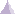# Solid PropertySolid property is a physical property assigned to a 3D part.

A solid property references a material assigned to this 3D part. A solid property is associative to the geometry this property points at.

The input and output characteristics are:

• Input:
• Material
• Output:
• Stress
• Strain
• Estimated error
• Stress Von Mises
• Elastic energy
• Elastic energy density
• Point force vector
• Pressure (optional)

The output characteristics can be expressed at the given positions in the element and in different axis systems:

 Position Axis System Characteristics Center of element Nodes of element Gauss point Face of element Global Local StressStrainEstimated errorStress Von MisesElastic energyElastic energy densityPoint force vectorPressure